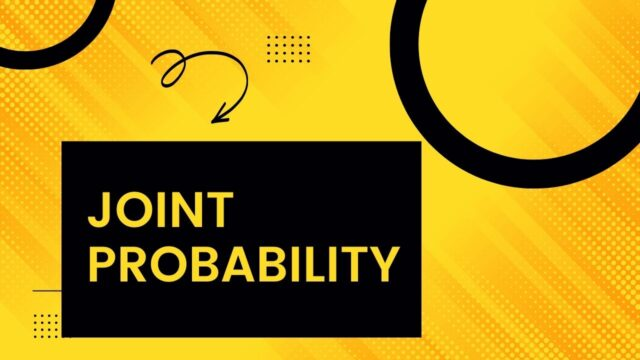# Joint Probability## What is joint probability

Joint probability is a statistical concept that refers to the likelihood of two events occurring together. For example, the joint probability of rolling a 6 on a die and flipping a coin landing on heads is 1/12. This is because there are a total of 12 possible outcomes (6 x 2), and only one of them is Rolling a 6 and Flipping a Coin Landing on Heads. Joint probability can be calculated by taking the product of the individual probabilities of each event occurring.

In the example above, the probability of Rolling a 6 is 1/6, and the probability of Flipping a Coin Landing on Heads is 1/2. So, the joint probability is 1/6 x 1/2 = 1/12. While joint probability can be used to calculate the likelihood of any two events occurring together, it is most commonly used in statistical models that involve multiple variables. These models can be used to predict things like the likelihood of two people with a certain genetic disposition developing a certain disease.

## How to calculate joint probability

To calculate joint probability, you need to know the individual probabilities of each event and the probability that the two events will occur together. The formula for joint probability is P(A and B) = P(A) x P(B). For example, if the probability of event A is 0.4 and the probability of event B is 0.6, then the joint probability of A and B occurring together is 0.4 x 0.6, or 0.24. Keep in mind that joint probability only applies when the two events are independent – that is, when one event does not affect the other event. If the events are dependent, then you would use conditional probability to calculate the likelihood of both events occurring.

## Examples of how to use joint probability

There are many ways to use joint probability, and the applications are really only limited by your imagination. Here are just a few examples:

• When planning an event, you can use joint probability to determine the likelihood that all the necessary conditions will be met (e.g., enough people will RSVP, the weather will be good, etc.).
• In marketing, joint probability can be used to predict the likelihood that a customer will purchase multiple products.
• In medicine, joint probability can be used to estimate the risk of developing certain diseases.
• In insurance, joint probability can be used to calculate premiums and policy limits.

These are just a few examples of how joint probability can be used. As you can see, it is a very versatile tool that can be applied in a wide variety of situations.

## Applications of joint probability

The concept of joint probability is used in a variety of settings, from weather forecasting to stock market analysis. In the most basic sense, joint probability is simply the likelihood that two events will occur simultaneously. For example, the joint probability of rain and snow could be used to forecast the likelihood of a winter storm. In Other applications, joint probability can be used to assess the risk of investments. For instance, if there is a low joint probability of recession and inflation, then this is generally considered to be a good time to invest in stocks. As another example, if the joint probability of two companies merging is high, then this may be a good time to buy shares in one or both companies. In each case, understanding joint probability can help make more informed decisions.

## Pitfalls to avoid when working with joint probabilities

There are several pitfalls to avoid when working with joint probabilities. First, it is important to remember that the marginal probability of each individual event must be less than or equal to 1. Second, the joint probability of two events must be less than or equal to the product of their individual marginal probabilities. Third, the joint probability of three events must be less than or equal to the product of their individual marginal probabilities. Finally, it is important to remember that the order in which the events occur does not affect the joint probability. These are just a few of the pitfalls to avoid when working with joint probabilities. By keeping these things in mind, you can avoid making mistakes that could lead to incorrect results.

## Tips for solving problems involving joint probabilities

There are a variety of problems that can be solved using joint probabilities, and there are a few different tips that can be useful when approaching these types of problems.

First, it can be helpful to draw a diagram of the situation. This can make it easier to visualize the different events and how they are related.

Second, it is important to identify all of the relevant information in the problem. This includes the probabilities of each individual event, as well as any information about dependence or independence between events. Once all of this information has been identified, the next step is to set up the equation that will be used to solve the problem. This equation should include all of the relevant information, such as the probabilities of each event and any conditional probabilities.

Finally, it is simply a matter of solving the equation and interpreting the results. With a little practice, solving problems involving joint probabilities can become quite straightforward.